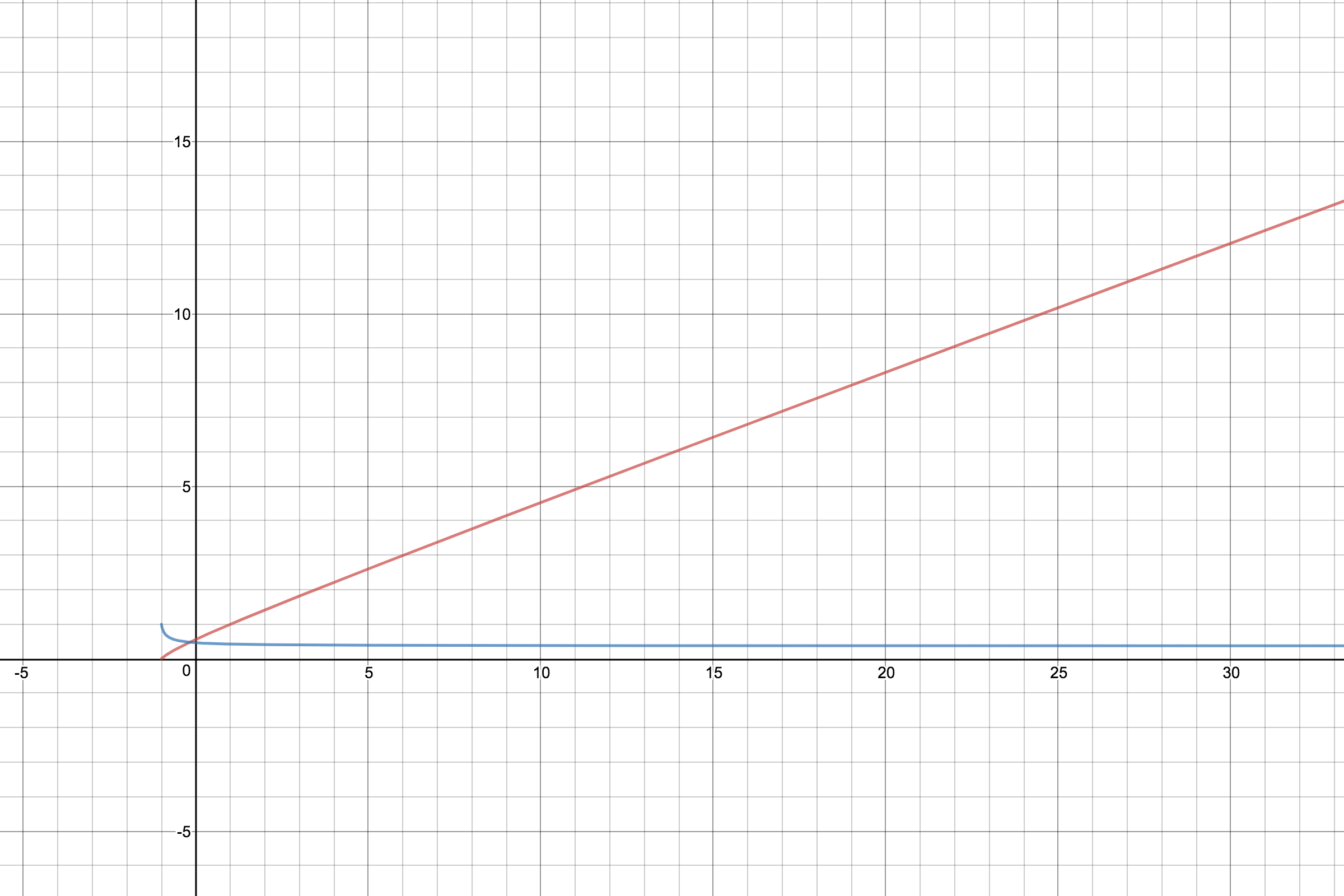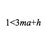# Calculus + Factorial = ?

Recently, I've been messing around with factorials (mainly because I was just told that $x! \approx (\frac{x}{e})^x\times\sqrt{2\pi x}$). I came across a couple calculus problems involving the function. Since I am very new to calculus, I figured I should ask the community on how to get the answers and what they are, not just by typing them into Wolfram Alpha and seeing what comes out.

Since $x!$ increases so rapidly, I decided taking the $x$ root of $x!$. This gave me the function, $\color{#D61F06}{f(x)=x!^{\frac{1}{x}}}$. That function is the red graph in the picture below.I noticed that, even though $f(0)$ is undefined (since$\frac{1}{0}$ is undefined), it still appears to have a value. That is my first problem: to find

$\lim_{x\rightarrow 0} \color{#D61F06}{f(x)}$

Next, I noticed that $\color{#D61F06}{f(x)}$ is not linear. However, it appeared to be linear, so I decided to graph the derivative, which gave me the function $\color{#3D99F6}{g(x)=\dfrac{\text{d}}{\text{d}x}~f(x)}$. This is the blue graph in the picture above. Since $\color{#D61F06}{f(x)}$ approached linearity (?), I knew that $\color{#3D99F6}{g(x)}$ must have a limit as it approached infinity. That leads me to my second problem: to find

$\lim_{x\rightarrow \infty} \color{#3D99F6}{g(x)}$

The graph is at this link.

NOTE: The domains of both functions stop before 171 because 171! is a massive number, to the point that most online calculators can't handle.Note by Blan Morrison
3 years, 4 months ago

This discussion board is a place to discuss our Daily Challenges and the math and science related to those challenges. Explanations are more than just a solution — they should explain the steps and thinking strategies that you used to obtain the solution. Comments should further the discussion of math and science.

When posting on Brilliant:

• Use the emojis to react to an explanation, whether you're congratulating a job well done , or just really confused .
• Ask specific questions about the challenge or the steps in somebody's explanation. Well-posed questions can add a lot to the discussion, but posting "I don't understand!" doesn't help anyone.
• Try to contribute something new to the discussion, whether it is an extension, generalization or other idea related to the challenge.

MarkdownAppears as
*italics* or _italics_ italics
**bold** or __bold__ bold
- bulleted- list
• bulleted
• list
1. numbered2. list
1. numbered
2. list
Note: you must add a full line of space before and after lists for them to show up correctly
paragraph 1paragraph 2

paragraph 1

paragraph 2

[example link](https://brilliant.org)example link
> This is a quote
This is a quote
    # I indented these lines
# 4 spaces, and now they show
# up as a code block.

print "hello world"
# I indented these lines
# 4 spaces, and now they show
# up as a code block.

print "hello world"
MathAppears as
Remember to wrap math in $$ ... $$ or $ ... $ to ensure proper formatting.
2 \times 3 $2 \times 3$
2^{34} $2^{34}$
a_{i-1} $a_{i-1}$
\frac{2}{3} $\frac{2}{3}$
\sqrt{2} $\sqrt{2}$
\sum_{i=1}^3 $\sum_{i=1}^3$
\sin \theta $\sin \theta$
\boxed{123} $\boxed{123}$

Sort by:

1. Stirling's formula is a good reference for the approximation. Note that this approximation holds for large values, and not necessarily for small values.

2. According to Wolfram Alpha, $\lim_{x\rightarrow 0 } f(x)$ is an interesting value.
It is not immediately apparant to me why this is true as yet.
Note: $\gamma$ is the Euler-Mascheroni constant.

3. Note that $f(x) \approx \frac{x}{e}$ for larget values. Hence, if there is any justice in the world (meaning that if the limit exists), it is most likely that \lim g(x) = \frac{1}[e} .

4. However, there is a slight flaw in your logic. Namely, the following statement is not true: "If $| f(x) - g(x) | < \epsilon$ for all $x$, then $\lim g'(x) = \lim f'(x)$.".
An extra condition will need to be added to arrive at "If $f(x) \approx g(x)$ (in some manner), then $\lim g'(x) = \lim f'(x)$."

Staff - 3 years, 4 months ago

$\displaystyle L=\lim_{n\to 0} (n!)^{\frac 1n}=\lim_{n\to 0} (\Gamma(n+1))^{\frac 1n}$ $\displaystyle L=\lim_{n\to 0} e^{\frac {\ln (\Gamma(n+1))}{n}}=e^{\lim_{n\to 0} \frac {\ln(\Gamma(n+1))}{n}}$

Using L'Hospital rule we get $\displaystyle L= e^{\lim_{n\to 0} \psi(n+1)}=e^{\psi(1)}$

And using $\psi(1)=-\gamma$ we get $L=e^{-\gamma}$

Where $\Gamma(.)$ is the Gamma function, $\psi(z)$ is the Digamma function and $\gamma$ is the Euler-Mascheroni constant

- 2 years, 4 months ago

I did some experimenting with factorials, here are some interested facts:

1. The limit as f(x) approaches 0 is equal to the negative root of the equation $x!=2$
2. The limit as g(x) approaches infinity is equal to this limit: $\large \lim_{x \to \infty}(x^{0.1}e^{-x})^{\frac{1}{x}}$ and also this limit: (x can be any postive real number) $\large \lim_{n \to 0}(x^{n}e^{-x})^{\frac{1}{x}}$

Those limits are derived from the gamma function, and the second one seem to evaluate to $\frac{1}{e}$

- 3 years, 3 months ago